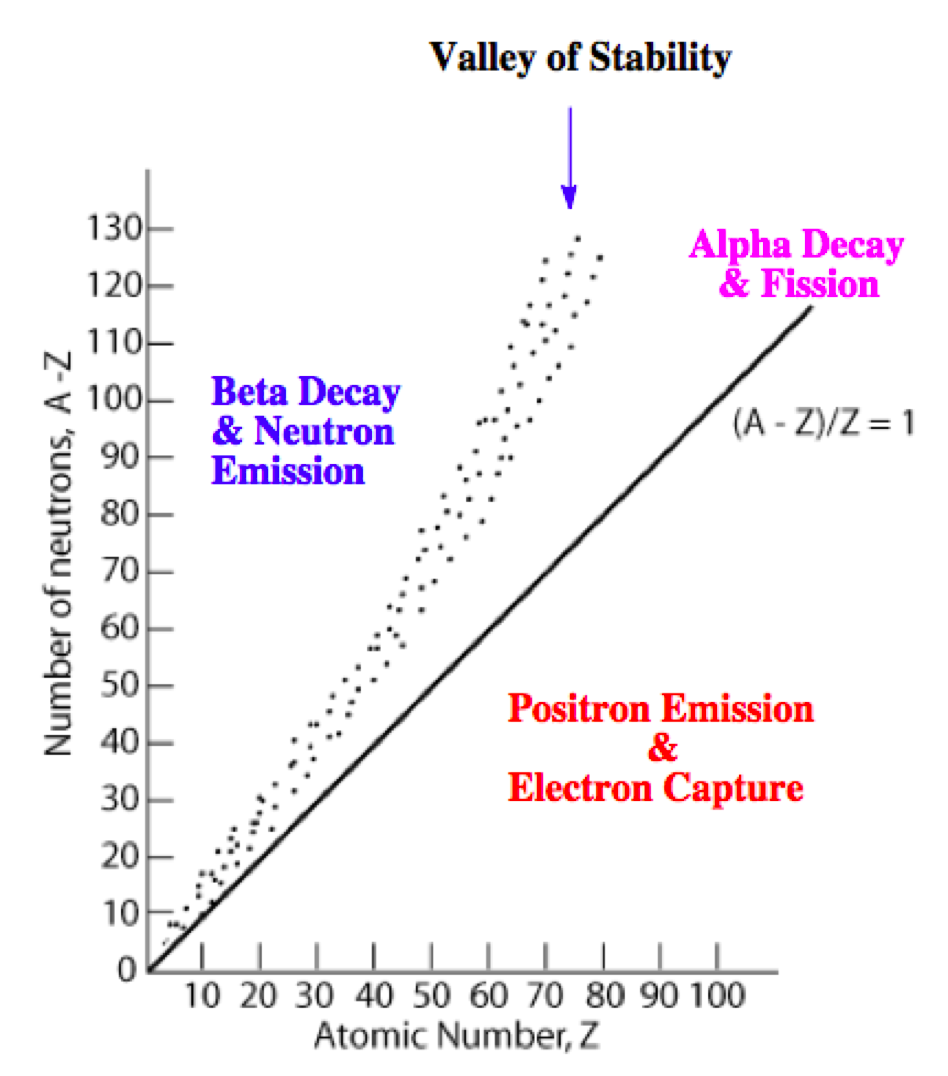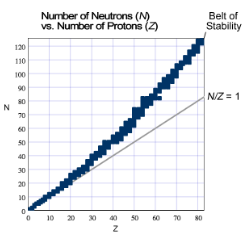# Problem: Which type of nuclear decay is the following radioactive isotope likely to undergo?All known stable isotopes are shown on the graph. The points form what is known as the "belt of stability". If a radioactive isotope is above the belt of stability, think about what type of decay would decrease its N/Z ratio. If an unstable isotope is below the belt of stability, think about what type of decay would increase its N/Z ratio. If a radioactive isotope is off the chart (Z &gt; 83), think about what type of decay would decrease Z the most.21385Ata. beta decayb. alpha decayc. positron emission or electron capture

###### FREE Expert Solution

The N/Z values for stable isotopes form the valley or band of stability:This means for isotopes:

• above the valley of stability: there too many neutrons in the isotope

Mode of decay: Either beta decay (0–1β) or neutron emission (10n; not in the choices)

• below the valley of stability: there too many protons in the isotope

Mode of decay: Either positron emission (01e) or electron capture (0–1e)

• with atomic mass ≥ 200 amu: there too many protons and neutrons in the isotope

Mode of decay: alpha decay (42α)

91% (394 ratings)###### Problem Details

Which type of nuclear decay is the following radioactive isotope likely to undergo?

All known stable isotopes are shown on the graph. The points form what is known as the "belt of stability". If a radioactive isotope is above the belt of stability, think about what type of decay would decrease its N/Z ratio. If an unstable isotope is below the belt of stability, think about what type of decay would increase its N/Z ratio. If a radioactive isotope is off the chart (Z > 83), think about what type of decay would decrease Z the most.

21385At

a. beta decay
b. alpha decay
c. positron emission or electron capture